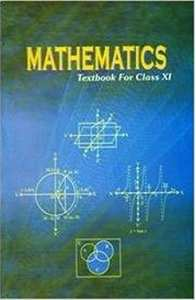CBSE (Commerce) Class 11CBSE
Share

NCERT solutions for Class 11 Mathematics chapter 5 - Complex Numbers and Quadratic Equations

Mathematics Textbook for Class 11

NCERT Mathematics Class 11Chapter 5: Complex Numbers and Quadratic Equations

NCERT Mathematics Class 11NCERT solutions for Class 11 Mathematics chapter 5 - Complex Numbers and Quadratic Equations

NCERT solutions for Class 11 Maths chapter 5 (Complex Numbers and Quadratic Equations) include all questions with solution and detail explanation. This will clear students doubts about any question and improve application skills while preparing for board exams. The detailed, step-by-step solutions will help you understand the concepts better and clear your confusions, if any. Shaalaa.com has the CBSE Mathematics Textbook for Class 11 solutions in a manner that help students grasp basic concepts better and faster.

Further, we at Shaalaa.com are providing such solutions so that students can prepare for written exams. NCERT textbook solutions can be a core help for self-study and acts as a perfect self-help guidance for students.

Concepts covered in Class 11 Mathematics chapter 5 Complex Numbers and Quadratic Equations are Argand Plane and Polar Representation, Quadratic Equations, Algebra of Complex Numbers - Equality, Algebraic Properties of Complex Numbers, Need for Complex Numbers, Square Root of a Complex Number, Complex Numbers, Algebra of Complex Numbers, The Modulus and the Conjugate of a Complex Number.

Using NCERT Class 11 solutions Complex Numbers and Quadratic Equations exercise by students are an easy way to prepare for the exams, as they involve solutions arranged chapter-wise also page wise. The questions involved in NCERT Solutions are important questions that can be asked in the final exam. Maximum students of CBSE Class 11 prefer NCERT Textbook Solutions to score more in exam.

Get the free view of chapter 5 Complex Numbers and Quadratic Equations Class 11 extra questions for Maths and can use Shaalaa.com to keep it handy for your exam preparation

S